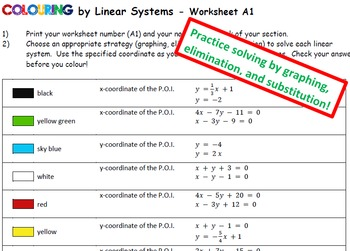# Colouring by Linear Systems, The Grinch (15 and 30-Sheet Collaborative Mosaics)8th - 12th
Subjects
Standards
Resource Type
Formats Included
• Zip
Pages
104 pages

### Description

Highly Engaging Holiday Fun! This task hits the intersection of collaborative motivation and individual accountability as students solve linear systems problems, both from equations and a variety of Christmas-themed application word problems. Each student's worksheet represents a small section of the large Grinch mosaic.

2 different mosaic sizes are included, to accommodate different class sizes. (15 and 30 sheet mosaics are included.)

Get this product as part of a MASSIVE linear systems bundle!

CHECK OUT THE PRODUCT PREVIEW to see exactly what type of problems students will be solving!

Find the Grinch in lots of topics!
Trig Ratios from Triangle Diagrams
Ratio Problems & Numeric Proportions
Volume and Surface Area Application Word Problems
Linear Systems - Equations, and Holiday Themed Application Word Problems
Sine & Cosine Law - Solve for Angle or Side
Linear Equations, 1 & 2 Step, multi-style bundle

Or, Get ALL my Christmas themed mosaics in one bundle for huge savings!

PRODUCT DETAILS:

• Each worksheet contain a randomized answer list at the bottom, allowing students to check their answers before colouring.
• All problems have (or round to) integer solutions for the two variables, although percentages, decimals or fractions may be involved in the process of solving.
• Each worksheet contains twelve problems

The first seven problems are given as two equations targeting these methods:
1) solve by graphing
2) solve by elimination
3) solve by graphing
4) solve by elimination
5) solve by substitution
6) mixed type, possibly involving fraction coefficients
7) solve by substitution

The last 5 are Christmas-themed word problems involving a variety of contexts:
• Money and Value (coin mixtures, ticket sales with different prices)
• Motion (distance, speed and time relationships)
• Mixtures (% concentration blends, candy mixtures with \$/pound)
• Word Equations (sum and difference of two numbers, written equations in words)

This task lends itself perfectly to small-groups, since each worksheet is different but structurally similar. Expect to overhear some rich mathematical dialog taking place! This is a challenging assignment, but the collaborative reward will drive them to the end goal.

A HANDY SOLUTION EXEMPLAR FILE IS INCLUDED! (See the product preview for an excerpt)

Leave the picture a secret or share it for motivation… it’s your call!

INCLUDED (.pdf and .docx everything):
• Complete class sets of worksheets for the 15, and 30-sheet mosaics
• Exemplar Solutions for every type of word problem contained on students' worksheets. Post this, or use it for examples during your lesson!
• Teaching Tips page for smooth implementation of this product
• Complete answer keys for all worksheets
• Colour image of the completed mosaic with coordinates to help you assemble the finished product

If you like this type of product, be sure the check out the whole Collaborative Math Mosaic directory, sorted by topic!

All my “Colouring by…” worksheets use standard pencil-crayon colours found in the Crayola 24 pack. For best results, use the exact colour name match, and have all student use the same colouring medium. Perhaps a class set of pencil crayons would be a fun math department investment!

CURRICULUM CONNECTION
CCSS:
•8.EE.C.8.c “Solve real-world and mathematical problems leading to two linear equations in two variables.”
• Also: 8.EE.C.8, 8.EE.C.8.a, HSA.REI.C.6, HSA.REI.C.5, High School: Modeling

• Analytic Geometry 1.1 “Solve systems of two linear equations involving two variables, using the algebraic method of substitution or elimination”
• Analytic Geometry 1.2 “Solve problems that arise from realistic situations described in words or represented by linear systems of two equations involving two variables, by choosing an appropriate algebraic or graphical method”

Feedback, suggestions, and frontline stories are always welcomed!

Thanks for checking this out! May your heart grow two sizes this year!
~CalfordMath (CalfordMath@live.ca)
Total Pages
104 pages
Included
Teaching Duration
2 days
Report this Resource to TpT
Reported resources will be reviewed by our team. Report this resource to let us know if this resource violates TpT’s content guidelines.

### Standards

to see state-specific standards (only available in the US).
Solve real-world and mathematical problems leading to two linear equations in two variables. For example, given coordinates for two pairs of points, determine whether the line through the first pair of points intersects the line through the second pair.
Understand that solutions to a system of two linear equations in two variables correspond to points of intersection of their graphs, because points of intersection satisfy both equations simultaneously.
Analyze and solve pairs of simultaneous linear equations.
Know and apply the properties of integer exponents to generate equivalent numerical expressions. For example, 3² × (3⁻⁵) = (3⁻³) = 1/3³ = 1/27.